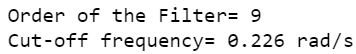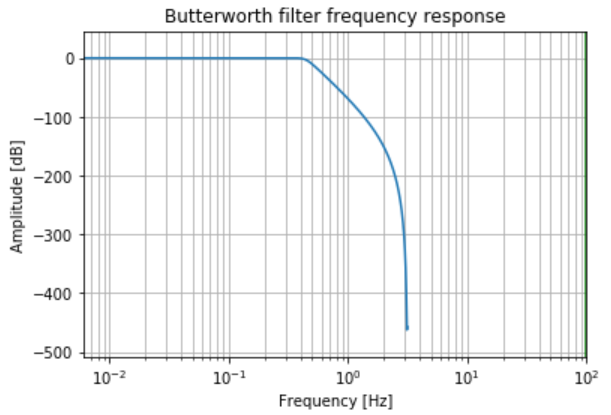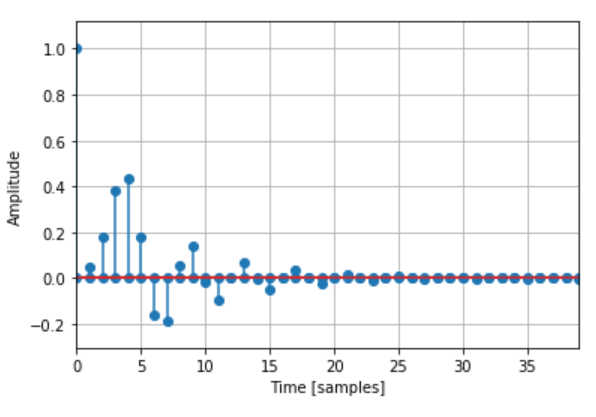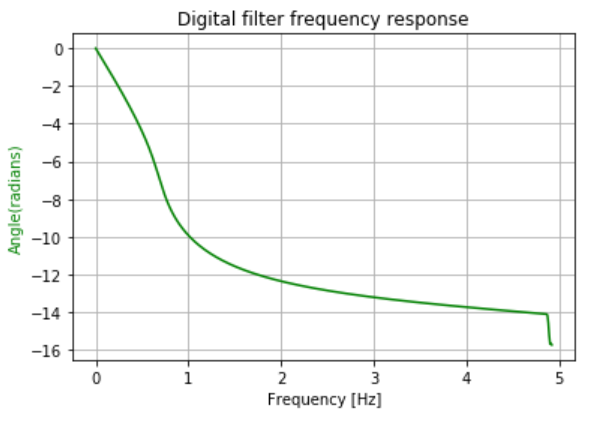# Digital Low Pass Butterworth Filter in Python

• Difficulty Level : Medium
• Last Updated : 08 Dec, 2020

In this article, we are going to discuss how to design a Digital Low Pass Butterworth Filter using Python. The Butterworth filter is a type of signal processing filter designed to have a frequency response as flat as possible in the pass band. Let us take the below specifications to design the filter and observe the Magnitude, Phase & Impulse Response of the Digital Butterworth Filter.

The specifications are as follows:

• Sampling rate of 40 kHz
• Pass band edge frequency of 4 kHz
• Stop band edge frequency of 8kHz
• Pass band ripple of 0.5 dB
• Minimum stop band attenuation of40 dB

We will plot the magnitude, phase, and impulse response of the filter.

### Step-by-step Approach:

Step 1: Importing all the necessary libraries.

## Python3

 `# import required modules``import` `numpy as np``import` `matplotlib.pyplot as plt``from` `scipy ``import` `signal``import` `math`

Step 2: Define variables with the given specifications of the filter.

## Python3

 `# Specifications of Filter`` ` ` ``# sampling frequency``f_sample ``=` `40000` ` ` `# pass band frequency``f_pass ``=` `4000`  ` ` `# stop band frequency``f_stop ``=` `8000`  ` ` `# pass band ripple``fs ``=` `0.5`` ` `# pass band freq in radian``wp ``=` `f_pass``/``(f_sample``/``2``)  `` ` `# stop band freq in radian``ws ``=` `f_stop``/``(f_sample``/``2``) `` ` `# Sampling Time``Td ``=` `1`  ` ` ` ``# pass band ripple``g_pass ``=` `0.5` ` ` `# stop band attenuation``g_stop ``=` `40`

Step3: Building the filter using signal.buttord function.

## Python3

 `# Conversion to prewrapped analog frequency``omega_p ``=` `(``2``/``Td)``*``np.tan(wp``/``2``)``omega_s ``=` `(``2``/``Td)``*``np.tan(ws``/``2``)`` ` ` ` `# Design of Filter using signal.buttord function``N, Wn ``=` `signal.buttord(omega_p, omega_s, g_pass, g_stop, analog``=``True``)`` ` ` ` `# Printing the values of order & cut-off frequency!``print``(``"Order of the Filter="``, N)  ``# N is the order``# Wn is the cut-off freq of the filter``print``(``"Cut-off frequency= {:.3f} rad/s "``.``format``(Wn))`` ` ` ` `# Conversion in Z-domain`` ` `# b is the numerator of the filter & a is the denominator``b, a ``=` `signal.butter(N, Wn, ``'low'``, ``True``)``z, p ``=` `signal.bilinear(b, a, fs)``# w is the freq in z-domain & h is the magnitude in z-domain``w, h ``=` `signal.freqz(z, p, ``512``)`

Output:Step 4: Plotting the Magnitude Response.

## Python3

 `# Magnitude Response``plt.semilogx(w, ``20``*``np.log10(``abs``(h)))``plt.xscale(``'log'``)``plt.title(``'Butterworth filter frequency response'``)``plt.xlabel(``'Frequency [Hz]'``)``plt.ylabel(``'Amplitude [dB]'``)``plt.margins(``0``, ``0.1``)``plt.grid(which``=``'both'``, axis``=``'both'``)``plt.axvline(``100``, color``=``'green'``)``plt.show()`

Output:Step 5: Plotting the Impulse Response.

## Python3

 `# Impulse Response``imp ``=` `signal.unit_impulse(``40``)``c, d ``=` `signal.butter(N, ``0.5``)``response ``=` `signal.lfilter(c, d, imp)`` ` `plt.stem(np.arange(``0``, ``40``), imp, use_line_collection``=``True``)``plt.stem(np.arange(``0``, ``40``), response, use_line_collection``=``True``)``plt.margins(``0``, ``0.1``)`` ` `plt.xlabel(``'Time [samples]'``)``plt.ylabel(``'Amplitude'``)``plt.grid(``True``)``plt.show()`

Output:Step 6: Plotting the Phase Response.

## Python3

 `# Phase Response``fig, ax1 ``=` `plt.subplots()`` ` `ax1.set_title(``'Digital filter frequency response'``)``ax1.set_ylabel(``'Angle(radians)'``, color``=``'g'``)``ax1.set_xlabel(``'Frequency [Hz]'``)`` ` `angles ``=` `np.unwrap(np.angle(h))`` ` `ax1.plot(w``/``2``*``np.pi, angles, ``'g'``)``ax1.grid()``ax1.axis(``'tight'``)``plt.show()`

Output:Below is the complete program based on the above approach:

## Python

 `# import required modules``import` `numpy as np``import` `matplotlib.pyplot as plt``from` `scipy ``import` `signal``import` `math`` ` ` ` `# Specifications of Filter`` ` ` ``# sampling frequency``f_sample ``=` `40000` ` ` `# pass band frequency``f_pass ``=` `4000`  ` ` `# stop band frequency``f_stop ``=` `8000`  ` ` `# pass band ripple``fs ``=` `0.5`` ` `# pass band freq in radian``wp ``=` `f_pass``/``(f_sample``/``2``)  `` ` `# stop band freq in radian``ws ``=` `f_stop``/``(f_sample``/``2``) `` ` `# Sampling Time``Td ``=` `1`  ` ` ` ``# pass band ripple``g_pass ``=` `0.5` ` ` `# stop band attenuation``g_stop ``=` `40`  ` ` ` ` `# Conversion to prewrapped analog frequency``omega_p ``=` `(``2``/``Td)``*``np.tan(wp``/``2``)``omega_s ``=` `(``2``/``Td)``*``np.tan(ws``/``2``)`` ` ` ` `# Design of Filter using signal.buttord function``N, Wn ``=` `signal.buttord(omega_p, omega_s, g_pass, g_stop, analog``=``True``)`` ` ` ` `# Printing the values of order & cut-off frequency!``print``(``"Order of the Filter="``, N)  ``# N is the order``# Wn is the cut-off freq of the filter``print``(``"Cut-off frequency= {:.3f} rad/s "``.``format``(Wn))`` ` ` ` `# Conversion in Z-domain`` ` `# b is the numerator of the filter & a is the denominator``b, a ``=` `signal.butter(N, Wn, ``'low'``, ``True``)``z, p ``=` `signal.bilinear(b, a, fs)``# w is the freq in z-domain & h is the magnitude in z-domain``w, h ``=` `signal.freqz(z, p, ``512``)`` ` ` ` `# Magnitude Response``plt.semilogx(w, ``20``*``np.log10(``abs``(h)))``plt.xscale(``'log'``)``plt.title(``'Butterworth filter frequency response'``)``plt.xlabel(``'Frequency [Hz]'``)``plt.ylabel(``'Amplitude [dB]'``)``plt.margins(``0``, ``0.1``)``plt.grid(which``=``'both'``, axis``=``'both'``)``plt.axvline(``100``, color``=``'green'``)``plt.show()`` ` ` ` `# Impulse Response``imp ``=` `signal.unit_impulse(``40``)``c, d ``=` `signal.butter(N, ``0.5``)``response ``=` `signal.lfilter(c, d, imp)``plt.stem(np.arange(``0``, ``40``), imp, use_line_collection``=``True``)``plt.stem(np.arange(``0``, ``40``), response, use_line_collection``=``True``)``plt.margins(``0``, ``0.1``)``plt.xlabel(``'Time [samples]'``)``plt.ylabel(``'Amplitude'``)``plt.grid(``True``)``plt.show()`` ` ` ` `# Phase Response``fig, ax1 ``=` `plt.subplots()``ax1.set_title(``'Digital filter frequency response'``)``ax1.set_ylabel(``'Angle(radians)'``, color``=``'g'``)``ax1.set_xlabel(``'Frequency [Hz]'``)``angles ``=` `np.unwrap(np.angle(h))``ax1.plot(w``/``2``*``np.pi, angles, ``'g'``)``ax1.grid()``ax1.axis(``'tight'``)``plt.show()`

Output:My Personal Notes arrow_drop_up